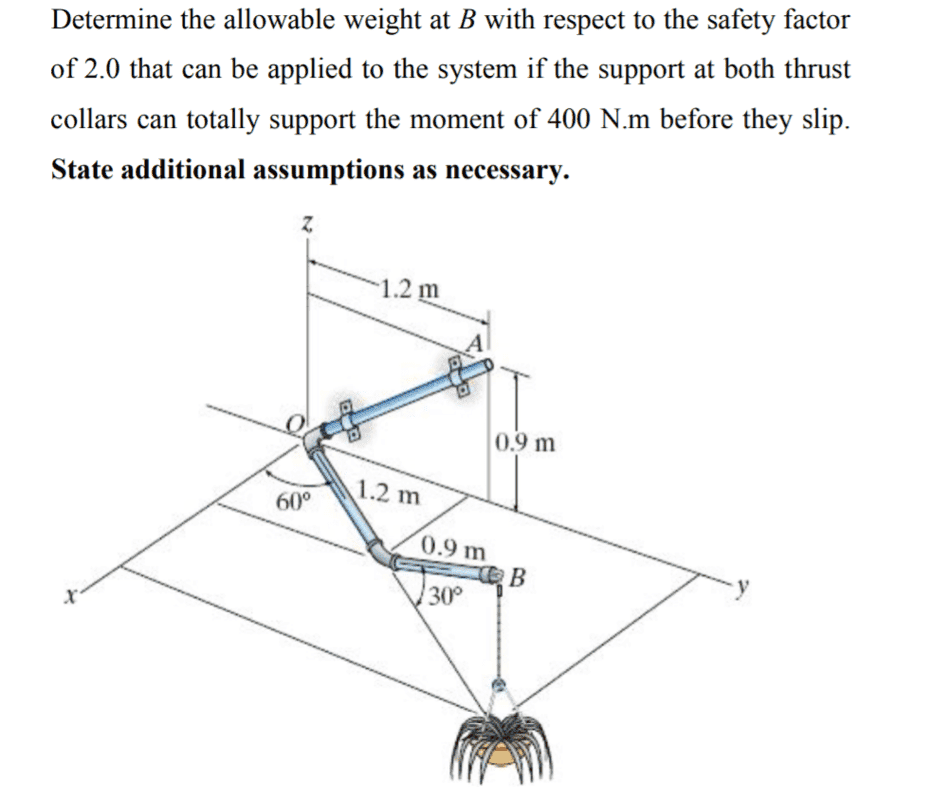# Problem on Equilibrium ( STATICS )

• lakuzamalakuzam
No, you need to solve for the entire system.In summary, the problem asks for the weight and force of the upper thrust collar, as well as the weight and force of the lower thrust collar, and the strength of the support reactions between them. It also asks for the horizontal radius from the O-B plane, the angle of the O-A axis to the horizontal, and the weight and force of the hanging weight.f

#### lakuzamalakuzamso i was wondering what assumptions needed to make this solvable and ' support at both thrust collars can totally support the moments of 400 Nm before they slip' means which axis of moment

Is this a homework problem?

There is a contradiction, in that the “thrust collars” must withstand a moment.
Thrust would be axial, moment would be about the axis.

Is that a moment of 400 N⋅m each, making 800 N⋅m, or a total of 400 N⋅m together ?

How much does the structure weigh, is that important ?

•lakuzamalakuzam
Yeah It's a homework problem.
I'm not sure what the problem means when it says 400 NM but from my understanding i think it is in OA axis with assumption that the system is properly aligned.
The structure weight is negligible.

The structure weight is negligible.
Is that a specified assumption, or an additional assumption ?

Yeah It's a homework problem.
I'm not sure what the problem means when it says 400 NM but from my understanding i think it is in OA axis with assumption that the system is properly aligned.
The structure weight is negligible.
but then there will be 9 unknowns ( Fx ,F//OA, Fperpendicular to OA, couple in OA axis for each of the thrust collar and W) with 3 scalar equation and 1 vector equation = 4 eq
so i think i will have to make further assumptions like there are no support reaction on the upper thrust collar and now there are 5 unknowns which isn't solvable

Last edited:
Is that a specified assumption, or an additional assumption ?